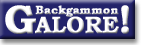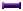Probability and StatisticsCalculating winning chances

 From: Douglas Zare Address: zare@math.columbia.edu Date: 23 June 2000 Subject: Re: How to calculate the ecspected winning-chances? Forum: rec.games.backgammon Google: 3953E045.652CE4FB@math.columbia.edu

```Erik Sřrensen wrote:

> The answer I want should take these factors as input:
> - The number of games played so far
> - The number of points actually won so far
>
> This should be the output:
> - By how much in percent am I favourite?
> - What is the uncertainty on this number?

There are a lot of factors and complications one could include, but I
won't. The following will give a rough method that is reasonably accurate
if you are winning or losing less than .5 points per game.

A fair estimate for your advantage in points per game is the number of
points you have won divided by the number of games. You could assume that
you have neither been lucky nor unlucky, and that the results display the
skill difference. Of course, don't do this if the number of games you have
played is 2 and you are up 38 points.

If this is your advantage, you next want to know the variance,
approximately the expected value of the square of the result of a game.
This is depends strongly on your playing styles. It might even be infinite
if you are too loose with the cube. I would guess that a variance of 6 is
plausible, but it might be higher or lower for you. Then the variance for n
games is 6n, e.g., 600 for 100 games. The standard deviation is the
square-root of the variance, and the standard deviation is what we want. If
you play a large number of games, there is about a 2/3 chance that the
result will be within 1 standard deviation of the average, and just over a
95% chance that the result will be within 2 standard deviations of the
average.

Suppose you have a .1 points per game advantage, a variance of 6, and you
play 100 games. The average result will be that you win 10 points, with a
variance of 600 -- a standard deviation of sqrt(600)~24.5. So 2/3 of the
time your score would be between -14 and +34, and 95% of the time it would
be between -39 and +59.

One can use this to give a different notion of confidence. Instead of
assuming that there was 0 net luck in the set of games from which you
will be 1 time in 40, or as bad as it will be 1 time in 40. 95% of the time
the correct value will be between the two estimates, but as you can see, it
takes much more than 100 games to get a decent window. If you won 10 points
in 100 games, this method would suggest that you have between a -.39
deficit and a +.59 advantage, so one should not actually be very surprised
if you score -50 in the next 100 games. It would probably mean that you
were lucky in the first 100 games, and unlucky in the second, but not
amazingly so.

Douglas Zare
```

### Probability and Statistics

Average game and match length  (JP White, Dec 2000)
Average luck of each roll  (Timothy Chow+, Mar 2013)
Average luck of each roll  (Jørn Thyssen+, Feb 2004)Calculating winning chances  (Douglas Zare, June 2000)
Chance of rolling x doubles in y rolls  (Raccoon+, July 2007)Chance of rolling x or more pips in y rolls  (Tom Keith, Feb 2004)Clumping of random numbers  (Gary Wong, Sept 1998)
Counting shots  (Koyunbaba+, June 2007)
Counting shots  (John Little+, Mar 2007)Distribution of points per game  (Roland Sutter, June 1999)
Distribution of points per game  (Stig Eide+, Sept 1995)Expected variation in points after a series of games  (Achim Müller+, Feb 1999)
How many games to decide who's better?  (Stephen Turner, Mar 1997)
How often is too often?  (Gary Wong, Oct 1998)
Losing after bearing off 14 checkers  (Daniel Murphy, July 1999)
Number of games per match  (Jason Lee+, Jan 2005)
Number of rolls to enter x checkers from bar  (Michael Depreli+, Mar 2011)
Visualizing odds  (Daithi, Mar 2011)From GammOnLineLong messageRecommended readingRecent addition

 Book Suggestions Books Cheating Chouettes Computer Dice Cube Handling Cube Handling in Races Equipment Etiquette Extreme Gammon Fun and frustration GNU Backgammon History Jellyfish Learning Luck versus Skill Magazines & E-zines Match Archives Match Equities Match Play Match Play at 2-away/2-away Miscellaneous Opening Rolls Pip Counting Play Sites Probability and Statistics Programming Propositions Puzzles Ratings Rollouts Rules Rulings Snowie Software Source Code Strategy--Backgames Strategy--Bearing Off Strategy--Checker play Terminology Theory Tournaments Uncategorized Variations